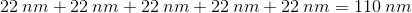## Example Questions

### Example Question #29 : Geometry

One side of a regular pentagon is. Find the perimeter of the pentagon.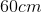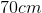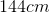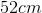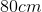Explanation:

In a regular pentagon, all of the sides are the same length.

The perimeter can then be found by summing allsides or multiplyingby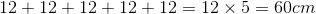### Example Question #30 : Geometry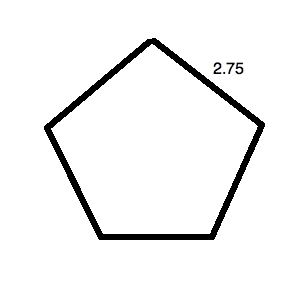What is the perimeter of the regular pentagon pictured above?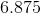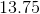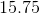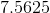Explanation:

The key word in this question is "regular." Since the pentagon is regular, all of its sides are equal. This means that it, in fact, looks like: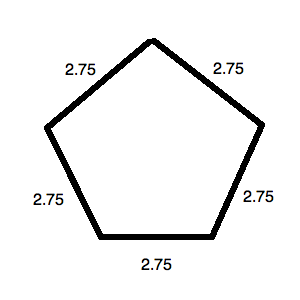The perimeter for this is simply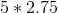or.

### Example Question #1 : How To Find The Perimeter Of A Pentagon

A regular pentagon has a side length of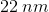. Find its perimeter.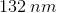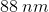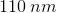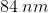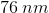Explanation:

Because we're told the pentagon is a regular polygon, this means that all of its sides are the same length. That is, the side lengths are congruent.

In order to solve for the perimeter, which is the sum of all sides, we can use a multiplication shortcut of: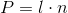, whereis perimeter,is length of the sides, andis the number of sides of the polygon.

Using the information given in the problem, the values can be substituted in.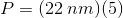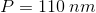Note that this math is the same as addingfive times: